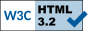# Table Driven Logic - A Simple Case

Requirement - A Sudoku solver, using logic (emulating human techniques) to solve a puzzle. There are (in this example) five different kinds of transformations that can performed upon the data set. We want to keep repeating the first transformation until there is no change; upon no change, we want to proceed to the second transformation. If the second transformation results in a change, we want to go back and start with the first transformation; otherwise we proceed onto the third transformation, and so on.

```
Do Until ( ¬ changed )
changed = test001()
If ( ¬ changed )
changed = test002()
If ( ¬ changed )
changed = test003()
If ( ¬ changed )
changed = test004()
If ( ¬ changed )
changed = test005()
EndIf
EndIf
EndIf
EndIf
EndDo
```

That's a lot of `If`s and `EndIf`s, and what would happen if we had two dozen transformations, or a thousand?

This whole top down structure can be implemented bottom up, through the use of Table Driven logic.

First, we create an array of Procedure Pointers:

```
d                 ds
d                                 *   ProcPtr Inz(*Null)
d @test@                  1     96*   ProcPtr Dim(6)
```

Note that there is one more entry in the array than there are transformations to execute.

Now, we define a pointer based procedure:

```
d test            pr             1n   ExtProc(test@)
```

and its basing pointer:

`d test@           s               *   ProcPtr`

and initialize tstCnt:

`d tstCnt          s              3s 0 Inz(%Elem(@test@))`

Now, we can implement the previous top down structure thusly;

```
DoU ( \$I = tstCnt );
\$I = 0;
DoU ( \$I = tstCnt Or test() );

\$I = \$I + 1;
test@ = @test@(\$I);

EndDo;
EndDo;
```

If we process all five transformation without change, then on the last iteration, `\$I` goes from five to six (5=>6), `test@` is loaded with a `*NULL`, and we fall out of our loop.

I have, in production code, implemented a Sarbanes-Oxley compliance report with a similar table driven structure. The process involves (roughly) fifteen different tests, and we want to fall out upon the first failure. The actual production mainline looks like:

```
\$I = 0;
DoU ( msgID <> *Blanks Or \$I = s100Cnt );
\$I = \$I + 1;
procProxy@ = @procProxy@(@s100(\$I));
procProxy();
EndDo;

cmtHndlr();
```

`msgID` is a global variable containing any error messages.

`s100Cnt` is the number of elements in `@s100`

`@s100` contains indexes to procedure pointers in `@procProxy@`; this allows us to change logic at run-time (see note) - if hard coding data is a bad thing, then it seems to reason hard coding logic is as well. This technique allow us to soft code logic.

Note: `@s100` is actually pointer based, allowing us to determine which of multiple arrays to use:

```d @s100           s              3s 0 Based(@s100@) Dim(15)

Select;
When ( system = SYSW And Not pmTESTMODE );
s100Cnt = %Elem(@S100W);
When ( system = SYSW And     pmTESTMODE );
s100Cnt = %Elem(@S100WT);
When ( system = SYSO And Not pmTESTMODE );